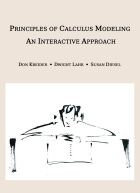# Material Detail## Calculus of the Dinner Table: Mathematical Modeling

Calculus students are presented with a write-pair-share activity that initially involves the construction of a model based on direct variation and later involves the use of calculus as a means by which to analyze the model. Suitable for either Calculus I or Calculus II students.

Note: This project has a sequel entitled Fundamental Theorem of Calculus: An Investigation (listed under Interactive Lectures) in which the Fundamental Theorem of Calculus is investigated via the constructed model.

Rate

#### Browse...

##### People who viewed this also viewed
••••##### Other materials like Calculus of the Dinner Table: Mathematical Modeling
••••